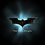# Amazing..!! How can this even be possible ??

Prove that:

1+2+3+4+5+6+.... up to infinity = -1/12 (negative of one-twelfth)

The above identity is used in many derivations of physics. I have a mathematical proof of the following, but please provide a logical one..Note by Rushi Rokad
7 years, 3 months ago

This discussion board is a place to discuss our Daily Challenges and the math and science related to those challenges. Explanations are more than just a solution — they should explain the steps and thinking strategies that you used to obtain the solution. Comments should further the discussion of math and science.

When posting on Brilliant:

• Use the emojis to react to an explanation, whether you're congratulating a job well done , or just really confused .
• Ask specific questions about the challenge or the steps in somebody's explanation. Well-posed questions can add a lot to the discussion, but posting "I don't understand!" doesn't help anyone.
• Try to contribute something new to the discussion, whether it is an extension, generalization or other idea related to the challenge.
• Stay on topic — we're all here to learn more about math and science, not to hear about your favorite get-rich-quick scheme or current world events.

MarkdownAppears as
*italics* or _italics_ italics
**bold** or __bold__ bold
- bulleted- list
• bulleted
• list
1. numbered2. list
1. numbered
2. list
Note: you must add a full line of space before and after lists for them to show up correctly
paragraph 1paragraph 2

paragraph 1

paragraph 2

[example link](https://brilliant.org)example link
> This is a quote
This is a quote
    # I indented these lines
# 4 spaces, and now they show
# up as a code block.

print "hello world"
# I indented these lines
# 4 spaces, and now they show
# up as a code block.

print "hello world"
MathAppears as
Remember to wrap math in $$ ... $$ or $ ... $ to ensure proper formatting.
2 \times 3 $2 \times 3$
2^{34} $2^{34}$
a_{i-1} $a_{i-1}$
\frac{2}{3} $\frac{2}{3}$
\sqrt{2} $\sqrt{2}$
\sum_{i=1}^3 $\sum_{i=1}^3$
\sin \theta $\sin \theta$
\boxed{123} $\boxed{123}$

## Comments

Sort by:

Top Newest

It took me 1 day to personally find out the mistakes that the university professors have done. Those people forgot the basic conditions required for treating a series as converging infinite series. In short this was just one stupid act of getting fame

- 7 years, 3 months ago

Log in to reply

are u sure???....could u show the proof???

- 7 years, 3 months ago

Log in to reply

Please see my reply to Achint Gupta..

- 7 years, 3 months ago

Log in to reply

Saw it...The numberphile video was amazing!!...Thanx!!

- 7 years, 2 months ago

Log in to reply

hows this possible???? I mean sum of positive natural numbers is negative !!!!!! How ???

- 7 years, 3 months ago

Log in to reply

Let S1 = 1 + -1 + -1 + 1 + -1 .....up to infinity = 1/2 (this can be proved by using the sum of infinite GP and even by simpler method. Let S2 = 1 -2 +3 -4 + 5 -6...up to infinity S2 + S2 = (1 -2 +3 -4....)+ (0 +1 -2 +3..) =S1 Therefore, 2 S2 = S1 = 1/2 Therefore, S2=1/4

Let S3 = 1 + 2 + 3 + 4 + 5.... Now, S3 - S2 = (1 + 2 + 3 +4...) -(1 -2 +3 -4..) = 4 + 8 + 12 +16..... = 4 (1+ 2 +3 +4...) = 4 (S3) Therefore, 3 (S3) = - S2 S3 = -1/12...

Sorry,I could not present it properly here.... For a better explanation , watch Numberphile on Youtube - https://www.youtube.com/watch?v=w-I6XTVZXww&list=TLB3KTqn9SZmDUThXfkDzwmDz8PRN0U-K-

- 7 years, 3 months ago

Log in to reply

This stuff can never ever be proved by "using sum of infinite GP or by any other simple maths ". But I thank u for that video link which shows us but i cant understand it as it involves some higher mathematics which i haven't yet studied

- 7 years, 3 months ago

Log in to reply

I got really something interesting for u After reading through the below link, u will find that such things aren't possible .

http://scientopia.org/blogs/goodmath/2014/01/17/bad-math-from-the-bad-astronomer/

- 7 years, 3 months ago

Log in to reply

Dat was Nice...

- 7 years, 3 months ago

Log in to reply

why can't we use sum of infinite GP..and take common ratio as -1 . we get the sum of the series as 1/2 .. ???

- 7 years, 3 months ago

Log in to reply

To use sum of infinite GP we have a condition that | r | < 1 , where r denotes the common ratio and " | | " is the modulus function

- 7 years, 3 months ago

Log in to reply

You can do it as such...

S1 = 1 + -1 +1 + -1 ...... S1 + S1 =( 1 + -1 + 1 + -1 + ...)+ ( 1 + -1 + 1 + -1...) 2 (S1) = 1 S1 = 1/2 ..Hence...

- 7 years, 3 months ago

Log in to reply

hey, that was grt proof.

- 7 years, 2 months ago

Log in to reply

Not mine..

- 7 years, 2 months ago

Log in to reply

×

Problem Loading...

Note Loading...

Set Loading...